# Counting Polyominoes

Category: Number Concepts and 3D Objects & 2D Shapes
Suitable for Grade Level: Elementary and Secondary

The Math in this Problem:

This classic problem has students working with a variety of polyominoes, studying their properties while performing all sorts of rotations with them. By studying these unique shapes, students can observe and comprehend the concepts of reflections and rotations by manipulating these various geometric forms.

Polyominoes are shapes that are made by gluing identical squares along their edges.

 A domino is a shape made from two squares glued along an edge: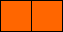A triomino is a shape made from three squares. There are only two different trominoes: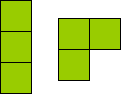A tetromino is a shape made from gluing four squares together. These two blue tetrominoes are actually the same because one can be rotated and flipped on its back to make the other.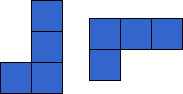These two tetrominoes are different. How many different tetrominoes can you find?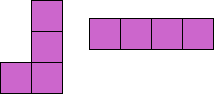A pentomino is a shape made from gluing five squares. The picture on The right shows two different pentominoes. How many different pentominoes are there?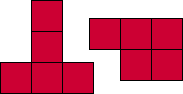#### Extensions:

If you are allowed to rotate a tetromino, but cannot flip it onto its back, how many different tetrominos are there? How many different pentominoes?

There is also no consensus about whether polynomials with holes should be considered polynomials. What is the smallest polynomial which has a hole? How did you define “hole”? What is the smallest size of polynomial which has 2, 3, 4, 5, 9 and 21 holes? Define “hole” in a different way and repeat. Make a generalization.

Define your own shapes using triangles or cubes or squares. How many are there?

Place all of the pentominoes into a 3 by 20 rectangle. There are only 2 different ways to do this if you exclude trivial rotations.